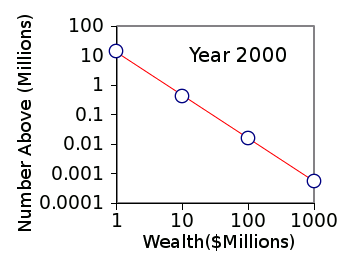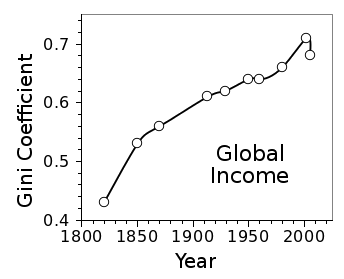### The Entropy of Nations

January 22, 2014

It's unlikely that my children will ever forget the unusual things their scientist father would say at the dinner table. One of the more notable of these was "people are like gas." Children find much humor in flatulence, so that was their first thought of what I was saying. I was actually expressing a well-known fact that an ensemble of people acts statistically like an ensemble of gas molecules.

The power of statistical mechanics is that its principles can be applied broadly. Since income inequality is presently a hot topic, I present the example in the following figure showing the partitioning of wealth between the many and the few in the United States for the year 2000.Distribution of US millionaires for the year 2000.

The data points for many scientists are far to the left of this graph.

(Data from ref. 2, graphed using Gnumeric.)

As the figure shows, it seems as if we're being guided by an invisible hand. Although it might not be possible to break away from the law expressed in the graph, we should take solace in the idea that the slope of the line, which sets the form of this exponential distribution, can be changed.

The quotation, "Prediction is very difficult, especially about the future," has been attributed to both Niels Bohr and Yogi Berra. The most useful function of statistics is to give us an idea of what will probably happen in the future. In a previous article (Cliodynamics, April 24, 2013), I wrote about cliodynamics, the mathematical modeling of historical trends with a view to predicting the future.

Entropy is a fundamental concept of thermodynamics, so much so that it's central to its second and third laws. Since there's an intimate connection between entropy and information, it's not unexpected that entropy is a useful idea outside of physics.

This connection between entropy and information is seen in the Boltzmann entropy formula, as follows:
S = kB ln(Ω),
where S is the system entropy, kB is the Boltzmann constant (1.38062 x 10-23 joule/kelvin), and ln(Ω) is the natural logarithm of the number of possible states that the system can have.

Entropy is used as a modeling tool, since the principle of maximum entropy states that the probability distribution that best fits your data is the one with the largest entropy. There are no simple examples of this, other than using the binary entropy function to decide that a coin toss should be 50:50. It's sufficient to note that there are more than 250 papers posted on arXiv with "Maximum Entropy" in the title, including a 111 page Ph.D. dissertation.

An open access paper entitled, "Global Inequality in Energy Consumption from 1980 to 2010," has just appeared in the journal, Entropy.[5-7] This paper, which is written by the physicists, Scott Lawrence, Qin Liu, and Victor M. Yakovenko of the University of Maryland (College Park) examines the global per capita consumption of energy in 2010. The partitioning of energy for individuals, as seen in the graph below, follows the same type of exponential distribution we see for the distribution of wealth in the graph above.Complementary cumulative probability distribution function for 2010 per capita global energy consumption.

The exponential fit on this log-linear scale is a straight line.

(Inset from fig. 3 of ref. 5.)

The authors attribute this exponential function to the globalization of the world economy, which brings the world closer to the state of maximum entropy. Their finding is that the top third of the world's population consumes two-thirds of its produced energy. Not surprisingly, a similar result is true for per-capita production of carbon dioxide emissions.[5-6] At least this distribution is not as bad as that for wealth in the United States. Here, the top richest one percent take home about 24 percent of income, and they hold about 30% of the wealth.

One measure of this distribution is the Gini coefficient, which is a measure of the inequality between the upper tiers and the lower tiers. The Gini coefficient is defined such that a value of zero indicates complete equality. A value of one indicates perfect inequality, typically expressed as one person having all the wealth. The Gini coefficient expressed in the above graph is 0.5.

As can be seen in the following graph, the Gini coefficient of individual income worldwide has been creeping up, a quantitative expression of increasing income inequality worldwide.Creeping Gini.

The Gini coefficient of global income has nearly doubled in the last two centuries.

(data from Wikipedia, graphed using Gnumeric.)### References:

Linked Keywords: Child; children; scientist; father; ideal gas; flatulence; society; ensemble of people; statistical mechanics; statistically; molecule; economic inequality; income inequality; wealth; United States; year 2000; millionaire; Gnumeric; invisible hand; slope; exponential distribution; Niels Bohr; Yogi Berra; statistics; forecasting; cliodynamics; mathematical model; history; historical; entropy; thermodynamics; second law of thermodynamics; third law of thermodynamics; information; physics; Boltzmann entropy formula; Boltzmann constant; joule; kelvin; natural logarithm; modeling; principle of maximum entropy; probability distribution; data; binary entropy function; arXiv; thesis; Ph.D. dissertation; open access journal; physicist; Scott Lawrence; Victor M. Yakovenko; University of Maryland (College Park); global per capita consumption of energy; complement; cumulative probability distribution function; semi-log plot; log-linear; economic globalization; globalization of the world economy; greenhouse gas; carbon dioxide emission; Gini coefficient; Income inequality in the United States; Walter the Farting Dog.